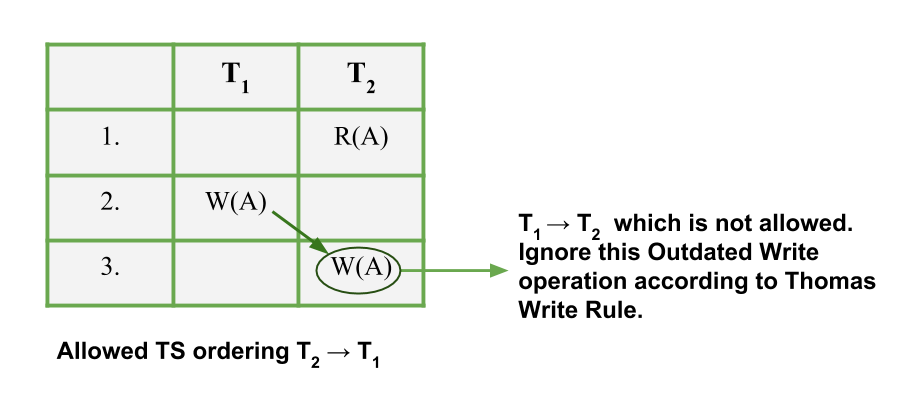# Thomas Write Rule in DBMS

Timestamp Ordering Protocol states that if Ri(X) and Wj(X) are conflicting operations then Ri (X) is processed before Wj(X) if and only if TS(Ti) < TS(Tj). Whenever a schedule does not follow serializablity order according to the Timestamp, user generally reject it and rollback the Transaction. Some operations on the other hand are harmless and can be allowed.

Thomas Write Rule allows such operations and is a modification on the Basic Timestamp Ordering protocol. In Thomas Write Rule user ignore outdated writes. Moreover, of all the Concurrency Protocols have been discussed, Concurrency is imposed on Schedules which are Conflict Serializable, in Thomas Write Rule, the most important improvement is user can achieve Concurrency with View Serializable schedules.

First let’s state what is Thomas Write Rule and then what are the modifications and improvements it succeeds over the Basic TO protocol.

Thomas Write Rule –
Thomas Write Rule does not enforce Conflict Serializablity but rejects fewer Write Operations by modifying the check Operations for W_item(X)

1. If R_TS(X) > TS(T), then abort and rollback T and reject the operation.
2. If W_TS(X) > TS(T), then don’t execute the Write Operation and continue processing. This is a case of Outdated or Obsolete Writes. Remember, outdated writes are ignored in Thomas Write Rule but a Transaction following Basic TO protocol will abort such a Transaction.
3. If neither the condition in 1 or 2 occurs, then and only then execute the W_item(X) operation of T and set W_TS(X) to TS(T)

Outdated Write Example –
The main update in Thomas Write Rule is ignoring the Obsolete Write Operations. This is done because some transaction with timestamp greater than TS(T) (i.e., a transaction after T in TS ordering) has already written the value of X. Hence, logically user can ignore the Write(X) operation of T which becomes obsolete. Let us see this through an example:

Suppose user has a schedule in which two transactions T1 and T2. Now, TS(T2) < TS(T1). This means T1 arrived after T2 and hence has a larger TS value than T2. This implies that serializablity of schedule allowed is T2 –> T1 . Consider the partial schedule given below:Image – Example of Outdated Write

Obsolete Writes are hence ignored in this rule which is in accordance to the 2nd protocol. It seems to be more logical as user skip an unnecessary procedure of restarting the entire transaction. This protocol is just a modification to Basic TO protocol.

Basic TO Protocol v/s Thomas Write Rule –
Suppose user has a schedule in which two transactions T1 and T2. Now, TS(T2) < TS(T1). This implies that serializablity of schedule allowed is T2 –> T1 . Consider the two protocols, let us see what types of Operation will be allowed and not allowed under them. Ri(A) implies Read and Wi(A) implies Write operation. Now, let us look at the types of partial schedules allowed in both Basic TO and Thomas Write Rule, you’ll understand the difference in operations of both the protocol. User distinguish in operations Allowed and Not Allowed in both of the Protocols.

Basic TO Protocol

• Not Allowed
• R1(X) W2(X)
• W1(X) R2(X)
• W1(X) W2(X)
• Allowed
• All operations where T2 occurs before T1.
• R1(X) R2(X)
Thomas Write Rule

• Not Allowed
• R1(X) W2(X)
• W1(X) R2(X)
• Allowed
• All operations where T2 occurs before T1.
• Outdated Writes: W1(X) W2(X)
• R1(X) R2(X)

Thus, from the above gist, this modification used in Thomas Write Rule in comparison to Basic TO protocol.

Reference: Database System Concepts, Fifth Edition [Silberschatz, Korth, Sudarshan], Chapter-16My Personal Notes arrow_drop_upCheck out this Author's contributed articles.

If you like GeeksforGeeks and would like to contribute, you can also write an article using contribute.geeksforgeeks.org or mail your article to contribute@geeksforgeeks.org. See your article appearing on the GeeksforGeeks main page and help other Geeks.

Please Improve this article if you find anything incorrect by clicking on the "Improve Article" button below.

Improved By : TamalMaity, S Das